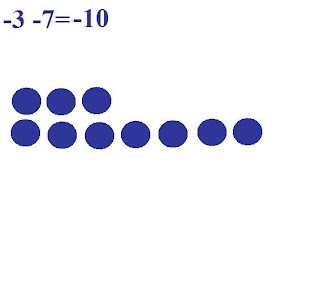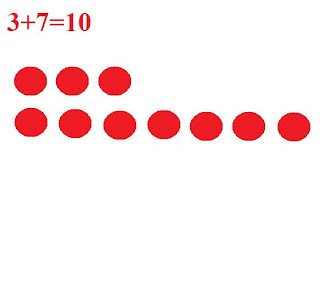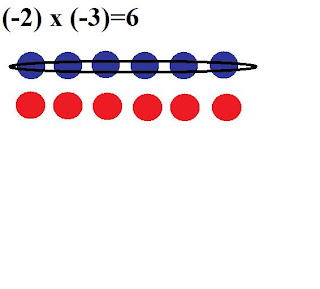## Monday, March 7, 2011

### Justins Great Big Book Of Integers

Chapter 1 Grade 7 Integer Review

Questions:
-6- (-4)=-2
-10+6=-4
6-7-2=1
14- (-3)=17Multiplying Integers

This question is saying to add two groups of positive 3's

This question is saying to add two groups of negative 3'sThis question is saying to take away 2 groups of positive 3's, I used zero pairs to do that.

This question is saying to take away 2 groups of negative 3's, I used zero pairs to do that.Sign Rule ( negative signs )

Even= When you have an even number of negative factors the product is Positive

Odd= When you have an odd number of negative factors the product is negative

Dividing Integers

If you have no negative or an even number of negative signs in a division question the quotient is positive
Ex ( 6 ÷ 3 =2 )

6÷3=2 <--- share 6 with 3 groups ( quotative division or sharing your total with groups )

6÷ 3=2 <--- how many groups of 3 are in 6 ( Partitive division or making parts )
When you have an odd number of ( - ) signs in a division question the quotient is negative

sign rule for division
6÷2=3 <---- how many 2's are in 6?

-6÷ (-2)= 3 <--- if there is an even amount of negatives the answer is positive

(-6)÷2= -3 <---- if there is an odd amount of negatives the answer is negative

6÷(-2)= -3 <---- if there is an odd amount of negatives the answer is negative

Order of Operations with Integers

(+5) x (-3) + (-6) ÷ (+3)
[(+5) x (-3)] + [(-6) ÷ (+3)]
-15+ (-2)= -17